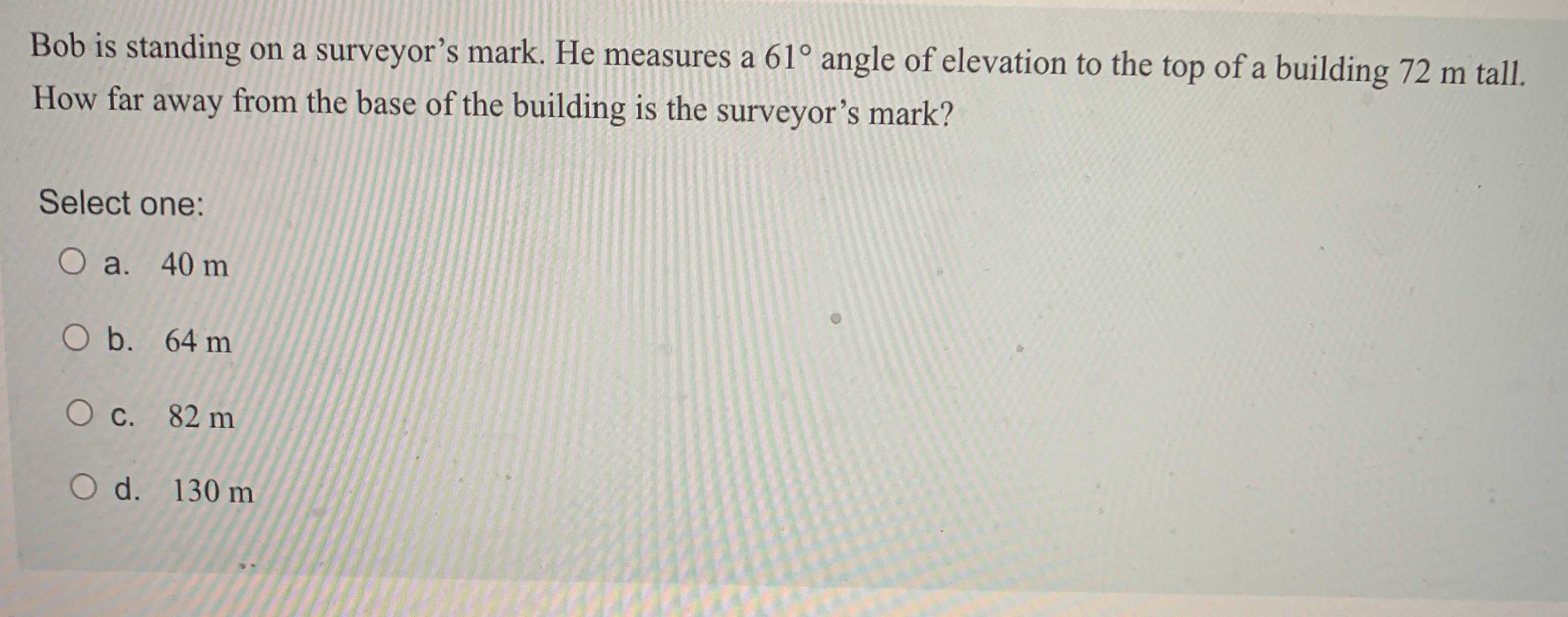### Still have math questions?

Trigonometry
QuestionBob is standing on a surveyor's mark. He measures a $$61 ^ { \circ }$$ angle of elevation to the top of a building $$72 m$$ tall. How far away from the base of the building is the surveyor's mark?

Select one:

a. $$40 m$$

b. $$64 m$$

c. $$82 m$$

d. $$130 m$$